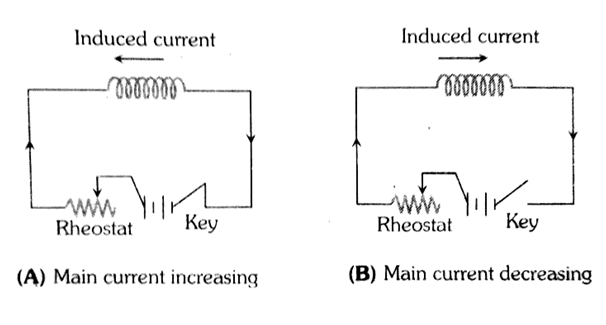Q

# Try this! A copper wire is wound on a wooden frame, whose shape is that of an equilateral triangle. If the linear dimension of each side of the frame is increased by factor of 3, keeping the number of turns of the coil per unit length of the frame the sam

A copper wire is wound on a wooden frame, whose shape is that of an equilateral triangle. If the linear dimension of each side of the frame is increased by factor of 3, keeping the number of turns of the coil per unit length of the frame the same, then the self inductance of the coil:

• Option 1)

decreases by factor of 9

• Option 2)

decreases by a factor of

• Option 3)

increases by factor of 3

• Option 4)

increases by  a factor of 27

Views

Self Inductance -

An emf is induced in the coil or the circuit which oppose the change that causes it. Which is also known back  emf.

- whereinlength of frame = 3a

No.of turn =0NLength of frame

No. of turn =

so

We known self-inductance

l=length of winding =d.N

where d=diameter of wire

Continued

putting l-dN in equation (3)

we set

Self-inductance

so self Inductance

So self inductance coil will increase by 27 factor.

Option 1)

decreases by factor of 9

Option 2)

decreases by a factor of

Option 3)

increases by factor of 3

Option 4)

increases by  a factor of 27

Exams
Articles
Questions